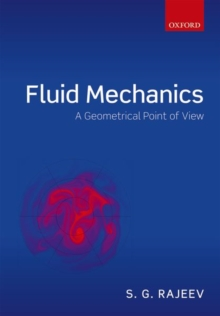# Fluid Mechanics : A Geometrical Point of View Hardback

#### Description

Fluid Mechanics: A Geometrical Point of View emphasizes general principles of physics illustrated by simple examples in fluid mechanics.

Advanced mathematics (e.g., Riemannian geometry and Lie groups) commonly used in other parts of theoretical physics (e.g.

General Relativity or High Energy Physics) are explained and applied to fluid mechanics. This follows on from the author's book Advanced Mechanics (Oxford University Press, 2013). After introducing the fundamental equations (Euler and Navier-Stokes), the book provides particular cases: ideal and viscous flows, shocks, boundary layers, instabilities, and transients. A restrained look at integrable systems (KdV) leads into a formulation of an ideal fluid as a hamiltonian system.

Arnold's deep idea, that the instability of a fluid can be understood using the curvature of the diffeomorphism group, will be explained.

Leray's work on regularity of Navier-Stokes solutions,and the modern developments arising from it, will be explained in language for physicists. Although this is a book on theoretical physics, readers will learn basic numerical methods: spectral and finite difference methods, geometric integrators for ordinary differential equations. Readers will take a deep dive into chaotic dynamics, using the Smale horse shoe as an example.

Aref's work on chaotic advection is explained. The book concludes with a self-contained introduction to renormalization, an idea from high energy physics which is expected to be useful in developing a theory ofturbulence.

#### Information

• Format: Hardback
• Pages: 272 pages, 30 grayscale line figures
• Publisher: Oxford University Press
• Publication Date:
• Category: Geometry
• ISBN: 9780198805021

£55.84

Free delivery within the UK